# pygimli.physics.ert#

Direct current electromagnetics

This package contains tools, modelling operators, and managers for Electrical Resistivity Tomography (ERT) & Induced polarization (IP)

## Overview#

Functions

 coverageERT coverageDCtrans( (object)S, (object)dd, (object)mm) -> object : createData(elecs[, schemeName]) Utility one-liner to create a BERT datafile createERTData(*args, **kwargs) createGeometricFactors(*args, **kwargs) createInversionMesh(data, **kwargs) Create default mesh for ERT inversion. drawERTData(ax, data[, vals]) Plot ERT data as pseudosection matrix (position over separation). estimateError(data[, absoluteError, ...]) Estimate error composed of an absolute and a relative part. generateDataPDF(data[, filename]) Generate a multi-page pdf showing all data properties. geometricFactor geometricFactors( (object)data [, (object)dim=3 [, (object)forceFlatEarth=False]]) -> object : geometricFactors(data [[, dim, forceFlatEarth]) Helper function to calculate configuration factors for a given DataContainerERT load(fileName[, verbose]) Shortcut to load ERT data. show(data[, vals]) Plot ERT data as pseudosection matrix (position over separation). showData(data[, vals]) Plot ERT data as pseudosection matrix (position over separation). showERTData(data[, vals]) Plot ERT data as pseudosection matrix (position over separation). simulate(mesh, scheme, res, **kwargs) Simulate an ERT measurement.

Classes

 DataContainer alias of DataContainerERT ERTManager([data]) ERT Manager. ERTModelling([sr, verbose]) Forward operator for Electrical Resistivity Tomography. ERTModellingReference(**kwargs) Reference implementation for 2.5D Electrical Resistivity Tomography. Manager alias of ERTManager VESManager(**kwargs) Vertical electrical sounding (VES) manager class. VESModelling([ab2, mn2]) Vertical Electrical Sounding (VES) forward operator.

## Functions#

pygimli.physics.ert.coverageERT()#

coverageDCtrans( (object)S, (object)dd, (object)mm) -> object :

C++ signature :

GIMLI::Vector<double> coverageDCtrans(GIMLI::MatrixBase,GIMLI::Vector<double>,GIMLI::Vector<double>)

pygimli.physics.ert.createData(elecs, schemeName='none', **kwargs)[source]#

Utility one-liner to create a BERT datafile

Parameters
• elecs (int | list[pos] | array(x)) – Number of electrodes or electrode positions or x-positions

• schemeName (str ['none']) – Name of the configuration. If you provide an unknown scheme name, all known schemes [‘wa’, ‘wb’, ‘pp’, ‘pd’, ‘dd’, ‘slm’, ‘hw’, ‘gr’] listed.

• **kwargs

Arguments that will be forwarded to the scheme generator.

• inversebool

interchange AB MN with MN AB

• reciprocitybool

interchange AB MN with BA NM

• spacingfloat 

electrode spacing in meters

• closedbool

Close the chain. Measure from the end of the array to the first electrode.

Returns

data

Return type

DataContainerERT

>>> import matplotlib.pyplot as plt
>>> from pygimli.physics import ert
>>>
>>> schemes = ['wa', 'wb', 'pp', 'pd', 'dd', 'slm', 'hw', 'gr']
>>> fig, ax = plt.subplots(3,3)
>>>
>>> for i, schemeName in enumerate(schemes):
...     s = ert.createData(elecs=41, schemeName=schemeName)
...     k = ert.geometricFactors(s)
...     _ = ert.show(s, vals=k, ax=ax.flat[i], label='k - ' + schemeName)
>>>
>>> plt.show()


(png, pdf)Examples using pygimli.physics.ert.createData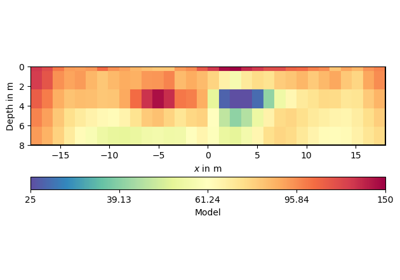2D ERT modeling and inversion

2D ERT modeling and inversion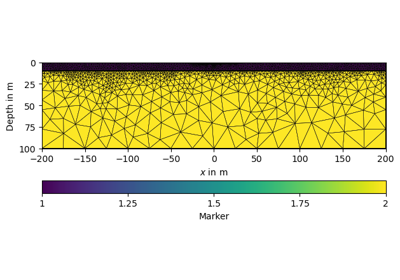2D FEM modelling on two-layer example

2D FEM modelling on two-layer example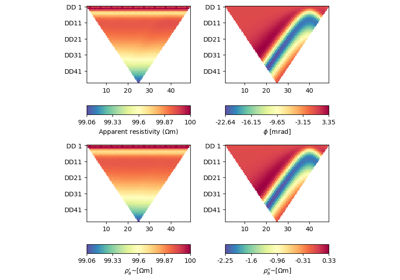Complex-valued electrical modeling

Complex-valued electrical modelingNaive complex-valued electrical inversion

Naive complex-valued electrical inversion
pygimli.physics.ert.createERTData(*args, **kwargs)#

Examples using pygimli.physics.ert.createERTData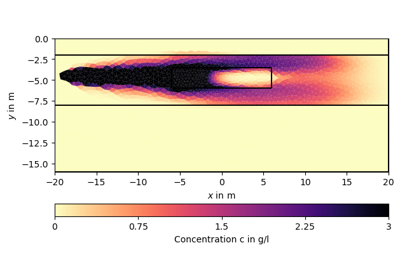Hydrogeophysical modeling

Hydrogeophysical modelingPetrophysical joint inversion

Petrophysical joint inversion
pygimli.physics.ert.createGeometricFactors(*args, **kwargs)#

Examples using pygimli.physics.ert.createGeometricFactorsERT field data with topography

ERT field data with topography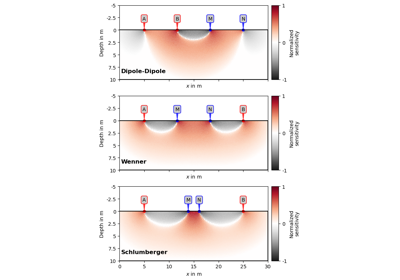Four-point sensitivities

Four-point sensitivitiesNaive complex-valued electrical inversion

Naive complex-valued electrical inversion
pygimli.physics.ert.createInversionMesh(data, **kwargs)[source]#

Create default mesh for ERT inversion.

Parameters

data (GIMLI::DataContainerERT) – Data Container needs at least sensors to define the geometry of the mesh.

:param Forwarded to pygimli.meshtools.createParaMesh:

Returns

mesh – Inversion mesh with default marker (1 for background, 2 parametric domain)

Return type

GIMLI::Mesh

pygimli.physics.ert.drawERTData(ax, data, vals=None, **kwargs)[source]#

Plot ERT data as pseudosection matrix (position over separation).

Parameters
• data (DataContainerERT) – data container with sensorPositions and a/b/m/n fields

• vals (iterable of data.size() [data['rhoa']]) – vector containing the vals to show

• ax (mpl.axis) – axis to plot, if not given a new figure is created

• cMin/cMax (float) – minimum/maximum color vals

• logScale (bool) – logarithmic colour scale [min(A)>0]

• label (string) – colorbar label

• **kwargs

• dxfloat

x-width of individual rectangles

• indinteger iterable or IVector

indices to limit display

• circularbool

Plot in polar coordinates when plotting via patchValMap

Returns

• ax – The used Axes

• cbar – The used Colorbar or None

pygimli.physics.ert.estimateError(data, absoluteError=0.001, relativeError=0.03, absoluteUError=None, absoluteCurrent=0.1)[source]#

Estimate error composed of an absolute and a relative part.

Parameters
• absoluteError (float [0.001]) – Absolute data error in Ohm m. Need ‘rhoa’ values in data.

• relativeError (float [0.03]) – relative error level in %/100

• absoluteUError (float [0.001]) – Absolute potential error in V. Need ‘u’ values in data. Or calculate them from ‘rhoa’, ‘k’ and absoluteCurrent if no ‘i’ is given

• absoluteCurrent (float [0.1]) – Current level in A for reconstruction for absolute potential V

Returns

error

Return type

Array

Examples using pygimli.physics.ert.estimateErrorERT field data with topography

ERT field data with topography2D crosshole ERT inversion

2D crosshole ERT inversion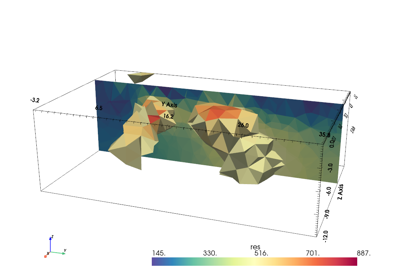3D surface ERT inversion

3D surface ERT inversion3D crosshole ERT inversion

3D crosshole ERT inversionIncorporating prior data into ERT inversion

Incorporating prior data into ERT inversion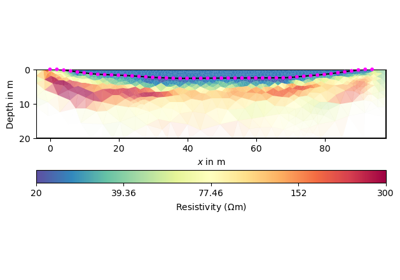Region-wise regularization

Region-wise regularization
pygimli.physics.ert.generateDataPDF(data, filename='data.pdf')[source]#

Generate a multi-page pdf showing all data properties.

pygimli.physics.ert.geometricFactor()#
geometricFactors( (object)data [, (object)dim=3 [, (object)forceFlatEarth=False]]) -> object :

Helper function to calculate configuration factors for a given DataContainerERT

C++ signature :

GIMLI::Vector<double> geometricFactors(GIMLI::DataContainerERT [,int=3 [,bool=False]])

pygimli.physics.ert.geometricFactors((object)data[, (object)dim=3[, (object)forceFlatEarth=False]]) object :#

Helper function to calculate configuration factors for a given DataContainerERT

C++ signature :

GIMLI::Vector<double> geometricFactors(GIMLI::DataContainerERT [,int=3 [,bool=False]])

Examples using pygimli.physics.ert.geometricFactors2D crosshole ERT inversion

2D crosshole ERT inversion3D surface ERT inversion

3D surface ERT inversion3D crosshole ERT inversion

3D crosshole ERT inversionIncorporating prior data into ERT inversion

Incorporating prior data into ERT inversionRegion-wise regularization

Region-wise regularization

Import Data and try to assume the file format. Additionally to unified data format we support the wide-spread res2dinv format as well as ASCII column files generated by the processing software of various instruments (ABEM LS, Syscal Pro, Resecs, ?)

If this fails, install pybert and use its auto importer pybert.importData.

Parameters

fileName (str) –

Returns

data

Return type

pg.DataContainer

pygimli.physics.ert.show(data, vals=None, **kwargs)#

Plot ERT data as pseudosection matrix (position over separation).

Creates figure, axis and draw a pseudosection.

Parameters
• data (BERT::DataContainerERT) –

• **kwargs

• axesmatplotlib.axes

Axes to plot into. Default is None and a new figure and axes are created.

• valsArray[nData]

Values to be plotted. Default is data[‘rhoa’].

Examples using pygimli.physics.ert.show2D ERT modeling and inversion

2D ERT modeling and inversionERT field data with topography

ERT field data with topographyIncorporating prior data into ERT inversion

Incorporating prior data into ERT inversionRegion-wise regularization

Region-wise regularization
pygimli.physics.ert.showData(data, vals=None, **kwargs)#

Plot ERT data as pseudosection matrix (position over separation).

Creates figure, axis and draw a pseudosection.

Parameters
• data (BERT::DataContainerERT) –

• **kwargs

• axesmatplotlib.axes

Axes to plot into. Default is None and a new figure and axes are created.

• valsArray[nData]

Values to be plotted. Default is data[‘rhoa’].

Examples using pygimli.physics.ert.showDataERT field data with topography

ERT field data with topographyPetrophysical joint inversion

Petrophysical joint inversion
pygimli.physics.ert.showERTData(data, vals=None, **kwargs)[source]#

Plot ERT data as pseudosection matrix (position over separation).

Creates figure, axis and draw a pseudosection.

Parameters
• data (BERT::DataContainerERT) –

• **kwargs

• axesmatplotlib.axes

Axes to plot into. Default is None and a new figure and axes are created.

• valsArray[nData]

Values to be plotted. Default is data[‘rhoa’].

Examples using pygimli.physics.ert.showERTDataComplex-valued electrical modeling

Complex-valued electrical modelingNaive complex-valued electrical inversion

Naive complex-valued electrical inversion
pygimli.physics.ert.simulate(mesh, scheme, res, **kwargs)[source]#

Simulate an ERT measurement.

Perform the forward task for a given mesh, resistivity distribution & measuring scheme and return data (apparent resistivity) or potentials.

For complex resistivity, the apparent resistivities is complex as well.

The forward operator itself only calculates potential values for the electrodes in the given data scheme. To calculate apparent resistivities, geometric factors (k) are needed. If there are no values k in the DataContainerERT scheme, the function tries to calculate them, either analytically or numerically by using a p2-refined version of the given mesh.

Parameters
• mesh (GIMLI::Mesh) – 2D or 3D Mesh to calculate for.

• res (float, array(mesh.cellCount()) | array(N, mesh.cellCount()) |) –

list Resistivity distribution for the given mesh cells can be: . float for homogeneous resistivity (e.g. 1.0) . single array of length mesh.cellCount() . matrix of N resistivity distributions of length mesh.cellCount() . resistivity map as [[regionMarker0, res0],

[regionMarker0, res1], …]

• scheme (GIMLI::DataContainerERT) – Data measurement scheme.

Keyword Arguments
• verbose (bool[False]) – Be verbose. Will override class settings.

• calcOnly (bool [False]) – Use fop.calculate instead of fop.response. Useful if you want to force the calculation of impedances for homogeneous models. No noise handling. Solution is put as token ‘u’ in the returned DataContainerERT.

• noiseLevel (float [0.0]) – add normally distributed noise based on scheme[‘err’] or on noiseLevel if error>0 is not contained

• noiseAbs (float [0.0]) – Absolute voltage error in V

• returnArray (bool [False]) – Returns an array of apparent resistivities instead of a DataContainerERT

• returnFields (bool [False]) – Returns a matrix of all potential values (per mesh nodes) for each injection electrodes.

Returns

• DataContainerERT | array(data.size()) | array(N, data.size()) |

• array(N, mesh.nodeCount()) – Data container with resulting apparent resistivity data and errors (if noiseLevel or noiseAbs is set). Optional returns a Matrix of rhoa values (for returnArray==True forces noiseLevel=0). In case of a complex valued resistivity model, phase values are returned in the DataContainerERT (see example below), or as an additionally returned array.

Examples

# >>> from pygimli.physics import ert # >>> import pygimli as pg # >>> import pygimli.meshtools as mt # >>> world = mt.createWorld(start=[-50, 0], end=[50, -50], # … layers=[-1, -5], worldMarker=True) # >>> scheme = ert.createData( # … elecs=pg.utils.grange(start=-10, end=10, n=21), # … schemeName=’dd’) # >>> for pos in scheme.sensorPositions(): # … _= world.createNode(pos) # … _= world.createNode(pos + [0.0, -0.1]) # >>> mesh = mt.createMesh(world, quality=34) # >>> rhomap = [ # … [1, 100. + 0j], # … [2, 50. + 0j], # … [3, 10.+ 0j], # … ] # >>> ert = pg.ERTManager() # >>> data = ert.simulate(mesh, res=rhomap, scheme=scheme, verbose=True) # >>> rhoa = data.get(‘rhoa’).array() # >>> phia = data.get(‘phia’).array()

Examples using pygimli.physics.ert.simulate2D ERT modeling and inversion

2D ERT modeling and inversion2D FEM modelling on two-layer example

2D FEM modelling on two-layer example3D modeling in a closed geometry

3D modeling in a closed geometryComplex-valued electrical modeling

Complex-valued electrical modelingNaive complex-valued electrical inversion

Naive complex-valued electrical inversionPetrophysical joint inversion

Petrophysical joint inversion

## Classes#

pygimli.physics.ert.DataContainer#

alias of DataContainerERT

class pygimli.physics.ert.ERTManager(data=None, **kwargs)[source]#

ERT Manager.

Method Manager for Electrical Resistivity Tomography (ERT)

__init__(data=None, **kwargs)[source]#

Create ERT Manager instance.

Parameters
• data (GIMLI::DataContainerERT | str) – You can initialize the Manager with data or give them a dataset when calling the inversion.

• useBert (*) – Use Bert forward operator instead of the reference implementation.

• sr (*) – Calculate with singularity removal technique. Recommended but needs the primary potential. For flat earth cases the primary potential will be calculated analytical. For domains with topography the primary potential will be calculated numerical using a p2 refined mesh or you provide primary potentials with setPrimPot.

checkData(data=None)[source]#

Return data from container.

THINKABOUT: Data will be changed, or should the manager keep a copy?

checkErrors(err, dataVals)[source]#

Check (estimate) and return relative error.

By default we assume ‘err’ are relative values.

coverage()[source]#

Coverage vector considering the logarithmic transformation.

createForwardOperator(**kwargs)[source]#

Create and choose forward operator.

createMesh(data=None, **kwargs)[source]#

Create default inversion mesh.

estimateError(data=None, **kwargs)[source]#

Estimate error composed of an absolute and a relative part.

Parameters
• absoluteError (float [0.001]) – Absolute data error in Ohm m. Need ‘rhoa’ values in data.

• relativeError (float [0.03]) – relative error level in %/100

• absoluteUError (float [0.001]) – Absolute potential error in V. Need ‘u’ values in data. Or calculate them from ‘rhoa’, ‘k’ and absoluteCurrent if no ‘i’ is given

• absoluteCurrent (float [0.1]) – Current level in A for reconstruction for absolute potential V

Returns

error

Return type

Array

Forwarded to pygimli.physics.ert.load

Parameters

fileName (str) – Filename for the data.

Returns

data

Return type

GIMLI::DataContainerERT

saveResult(folder=None, size=(16, 10), **kwargs)[source]#

Save all results in the specified folder.

Saved items are:

Inverted profile Resistivity vector Coverage vector Standardized coverage vector Mesh (bms and vtk with results)

setPrimPot(pot)[source]#

Set primary potential from external is not supported anymore.

setSingularityRemoval(sr=True)[source]#

Turn singularity removal on or off.

showMisfit(errorWeighted=False, **kwargs)[source]#

Show relative or error-weighted data misfit.

showModel(model=None, elecs=True, ax=None, **kwargs)[source]#

Show the last inversion result.

Parameters
• ax (mpl axes) – Axes object to draw into. Create a new if its not given.

• model (iterable [None]) – Model values to be draw. Default is self.model from the last run

Return type

ax, cbar

simulate(mesh, scheme, res, **kwargs)[source]#

Simulate an ERT measurement.

Perform the forward task for a given mesh, resistivity distribution & measuring scheme and return data (apparent resistivity) or potentials.

For complex resistivity, the apparent resistivities is complex as well.

The forward operator itself only calculates potential values for the electrodes in the given data scheme. To calculate apparent resistivities, geometric factors (k) are needed. If there are no values k in the DataContainerERT scheme, the function tries to calculate them, either analytically or numerically by using a p2-refined version of the given mesh.

Parameters
• mesh (GIMLI::Mesh) – 2D or 3D Mesh to calculate for.

• res (float, array(mesh.cellCount()) | array(N, mesh.cellCount()) |) –

list Resistivity distribution for the given mesh cells can be: . float for homogeneous resistivity (e.g. 1.0) . single array of length mesh.cellCount() . matrix of N resistivity distributions of length mesh.cellCount() . resistivity map as [[regionMarker0, res0],

[regionMarker0, res1], …]

• scheme (GIMLI::DataContainerERT) – Data measurement scheme.

Keyword Arguments
• verbose (bool[False]) – Be verbose. Will override class settings.

• calcOnly (bool [False]) – Use fop.calculate instead of fop.response. Useful if you want to force the calculation of impedances for homogeneous models. No noise handling. Solution is put as token ‘u’ in the returned DataContainerERT.

• noiseLevel (float [0.0]) – add normally distributed noise based on scheme[‘err’] or on noiseLevel if error>0 is not contained

• noiseAbs (float [0.0]) – Absolute voltage error in V

• returnArray (bool [False]) – Returns an array of apparent resistivities instead of a DataContainerERT

• returnFields (bool [False]) – Returns a matrix of all potential values (per mesh nodes) for each injection electrodes.

Returns

• DataContainerERT | array(data.size()) | array(N, data.size()) |

• array(N, mesh.nodeCount()) – Data container with resulting apparent resistivity data and errors (if noiseLevel or noiseAbs is set). Optional returns a Matrix of rhoa values (for returnArray==True forces noiseLevel=0). In case of a complex valued resistivity model, phase values are returned in the DataContainerERT (see example below), or as an additionally returned array.

Examples

# >>> from pygimli.physics import ert # >>> import pygimli as pg # >>> import pygimli.meshtools as mt # >>> world = mt.createWorld(start=[-50, 0], end=[50, -50], # … layers=[-1, -5], worldMarker=True) # >>> scheme = ert.createData( # … elecs=pg.utils.grange(start=-10, end=10, n=21), # … schemeName=’dd’) # >>> for pos in scheme.sensorPositions(): # … _= world.createNode(pos) # … _= world.createNode(pos + [0.0, -0.1]) # >>> mesh = mt.createMesh(world, quality=34) # >>> rhomap = [ # … [1, 100. + 0j], # … [2, 50. + 0j], # … [3, 10.+ 0j], # … ] # >>> data = ert.simulate(mesh, res=rhomap, scheme=scheme, verbose=1) # >>> rhoa = data.get(‘rhoa’).array() # >>> phia = data.get(‘phia’).array()

standardizedCoverage(threshold=0.01)[source]#

Return standardized coverage vector (0|1) using thresholding.

class pygimli.physics.ert.ERTModelling(sr=True, verbose=False)[source]#

Bases: ERTModellingBase

Forward operator for Electrical Resistivity Tomography.

Note

Convention for complex resistiviy inversion: We want to use logarithm transformation for the imaginary part of model so we need the startmodel to have positive imaginary parts. The sign is flipped back to physical correct assumption before we call the response function. The Jacobian is calculated with negative imaginary parts and will be a conjugated complex block matrix for further calulations.

__init__(sr=True, verbose=False)[source]#

Initialize.

Variables
• fop (pg.frameworks.Modelling) –

• data (pg.DataContainer) –

• modelTrans ([pg.trans.TransLog()]) –

Parameters

**kwargs – fop : Modelling

createJacobian(mod)[source]#

Compute Jacobian matrix and store but not return.

createStartModel(dataVals)[source]#

Create Starting model for ERT inversion.

flipImagPart(v)[source]#

Flip imaginary port (convention).

response(mod)[source]#

Forward response (apparent resistivity).

setDataPost(data)[source]#

Set data (at a later stage).

setDefaultBackground()[source]#

Set the default background behaviour.

setMeshPost(mesh)[source]#

Set mesh (at a later stage).

setVerbose(v)[source]#

Set verbosity.

class pygimli.physics.ert.ERTModellingReference(**kwargs)[source]#

Bases: ERTModellingBase

Reference implementation for 2.5D Electrical Resistivity Tomography.

__init__(**kwargs)[source]#

Initialize.

Variables
• fop (pg.frameworks.Modelling) –

• data (pg.DataContainer) –

• modelTrans ([pg.trans.TransLog()]) –

Parameters

**kwargs – fop : Modelling

calcGeometricFactor(data)[source]#

Calculate geometry factors for a given dataset.

createJacobian(model)[source]#

Create Jacobian matrix for model and store it in self.jacobian().

createRHS(mesh, elecs)[source]#

Create right-hand-side vector.

getIntegrationWeights(rMin, rMax)[source]#

TODO WRITEME.

mixedBC(boundary, userData)[source]#

Apply mixed boundary conditions.

pointSource(cell, f, userData)[source]#

Define function for the current source term.

$$\delta(x-pos), \int f(x) \delta(x-pos)=f(pos)=N(pos)$$

Right hand side entries will be shape functions(pos)

response(model)[source]#

Solve forward task and return apparent resistivity for self.mesh.

uAnalytical(p, sourcePos, k)[source]#

Calculate analytical potential for homogeneous halfspace.

For sigma = 1 [S m]

pygimli.physics.ert.Manager#

alias of ERTManager

class pygimli.physics.ert.VESManager(**kwargs)[source]#

Vertical electrical sounding (VES) manager class.

>>> import numpy as np
>>> import pygimli as pg
>>> from pygimli.physics import VESManager
>>> ab2 = np.logspace(np.log10(1.5), np.log10(100), 32)
>>> mn2 = 1.0
>>> # 3 layer with 100, 500 and 20 Ohmm
>>> # and layer thickness of 4, 6, 10 m
>>> # over a Halfspace of 800 Ohmm
>>> synthModel = pg.cat([4., 6., 10.], [100., 5., 20., 800.])
>>> ves = VESManager()
>>> ra, err = ves.simulate(synthModel, ab2=ab2, mn2=mn2, noiseLevel=0.01)
>>> ax = ves.showData(ra, error=err)
>>> model = ves.invert(ra, err, nLayers=4, showProgress=0, verbose=0)
>>> ax, _ = ves.showModel(synthModel)
>>> _ = ves.showResult(ax=ax)(png, pdf)#(png, pdf)#

__init__(**kwargs)[source]#

Initialize instance.

Parameters

complex (bool) – Accept complex resistivities.

Variables

complex (bool) – Accept complex resistivities.

property complex#

Return whether the computations are complex.

createForwardOperator(**kwargs)[source]#

Create Forward Operator.

Create Forward Operator based on complex attribute.

exportData(fileName, data=None, error=None)[source]#

Export data into simple ascii matrix.

Usefull?

invert(data=None, err=None, ab2=None, mn2=None, **kwargs)[source]#

Invert measured data.

Parameters
• data (iterable) – data vector

• err (iterable) – error vector

• ab2 (iterable) – AB/2 vector (otherwise taken from data)

• mn2 (iterable) – MN/2 vector (otherwise taken from data)

Keyword Arguments

**kwargs – Additional kwargs inherited from %(MethodManager1d.invert) and %(Inversion.run)

Returns

model – inversion result

Return type

pg.Vector

preErrorCheck(err, dataVals=None)[source]#

Fct to be called before the validity check of the error values.

simulate(model, ab2=None, mn2=None, **kwargs)[source]#

Simulate measurement data.

class pygimli.physics.ert.VESModelling(ab2=None, mn2=None, **kwargs)[source]#

Vertical Electrical Sounding (VES) forward operator.

Variables
• ab2 – Half distance between the current electrodes A and B.

• mn2 – Half distance between the potential electrodes M and N. Only used for input (feeding am etc.) or plotting.

• am – Part of data basis. Distances between A and M electrodes. A is first current, M is first potential electrode.

• bm – Part of data basis. Distances between B and M electrodes. B is second current, M is first potential electrode.

• an – Part of data basis. Distances between A and N electrodes. A is first current, N is second potential electrode.

• bn – Part of data basis. Distances between B and N electrodes. B is second current, N is second potential electrode.

__init__(ab2=None, mn2=None, **kwargs)[source]#

Initialize with distances.

createStartModel(rhoa)[source]#

Create starting model.

drawData(ax, data, error=None, label=None, **kwargs)[source]#

Draw modeled apparent resistivity data.

Parameters
• ax (axes) – Matplotlib axes object to draw into.

• data (iterable) – Apparent resistivity values to draw.

• error (iterable [None]) – Adds an error bar if you have error values.

• label (str ['$rho_a$']) – Set legend label for the amplitude.

• ab2 (iterable) – Override ab2 that fits data size.

• mn2 (iterable) – Override mn2 that fits data size.

• plot (function name) – Matplotlib plot function, e.g., plot, loglog, semilogx or semilogy

drawModel(ax, model, **kwargs)[source]#

Draw model as 1D block model.

response(par)[source]#

Model response.

response_mt(par, i=0)[source]#